Study of mathematics online.
Study math with us and make sure that "Mathematics is easy!"

Surface Area Formulas for geometric shapes

The surface area
is the sum of areas of all shapes that cover the surface of the geometric figure.

Surface Area of a Cube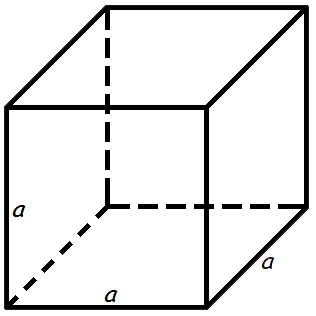The surface area of a cube is 6 times one of the sides squared.

Surface area formula of a cube:

A = 6 a2
where A - surface area of the cube,
a - the length of the side of each edge of the cube.

Surface Area of a Rectangular Prism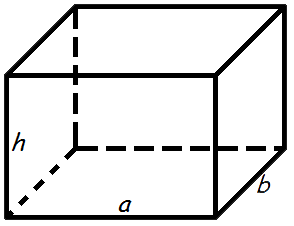The surface area of a rectangular prism is the area of the six rectangles that cover it.

Surface area formula of a rectangular prism:

A = 2(a · b + a · h + b · h)
where A - surface area of the rectangular prism,
a - length,
b - width,
h - height.

Surface Area of a Cylinder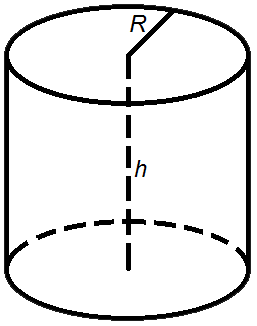Area of the cylinder side is the perimeter of top multiplied by height.

Area formula of the cylinder side:

A = 2 π R h

The surface area of a cylinder is the sum of the area of the cylinder side and two areas of top.

The formula for the surface area of a cylinder:

A = 2 π R h + 2 π R 2 = 2 π R(R + h)
where A - area,
R - the radius of the top,
h - the height of the cylinder,
π = 3.141592.

Surface area of a Right Circular Cone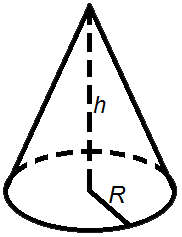The lateral surface area of a cone is slant times radius multiplied by π.

The formula for the lateral surface area of a cone:

A = π R l

The surface area of a cone is the sum of the area of the base (circular bottom) and the lateral surface area.

Surface area formula of a cone:

A = π R2 + π R l = π R (R + l)
where A - area,
R - radius of the base,
l - slant height,
π = 3.141592.

Surface Area of a Sphere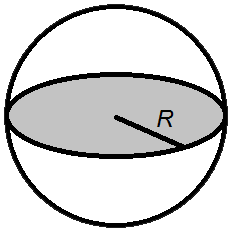Surface area formulas of a sphere:

• The surface area formulas of a sphere is 4 times radius squared multiplied by π.

A = 4 π R2
• The surface area formulas of a sphere is diameter squared multiplied by π.

A = π D2
where A - the surface area of a sphere,